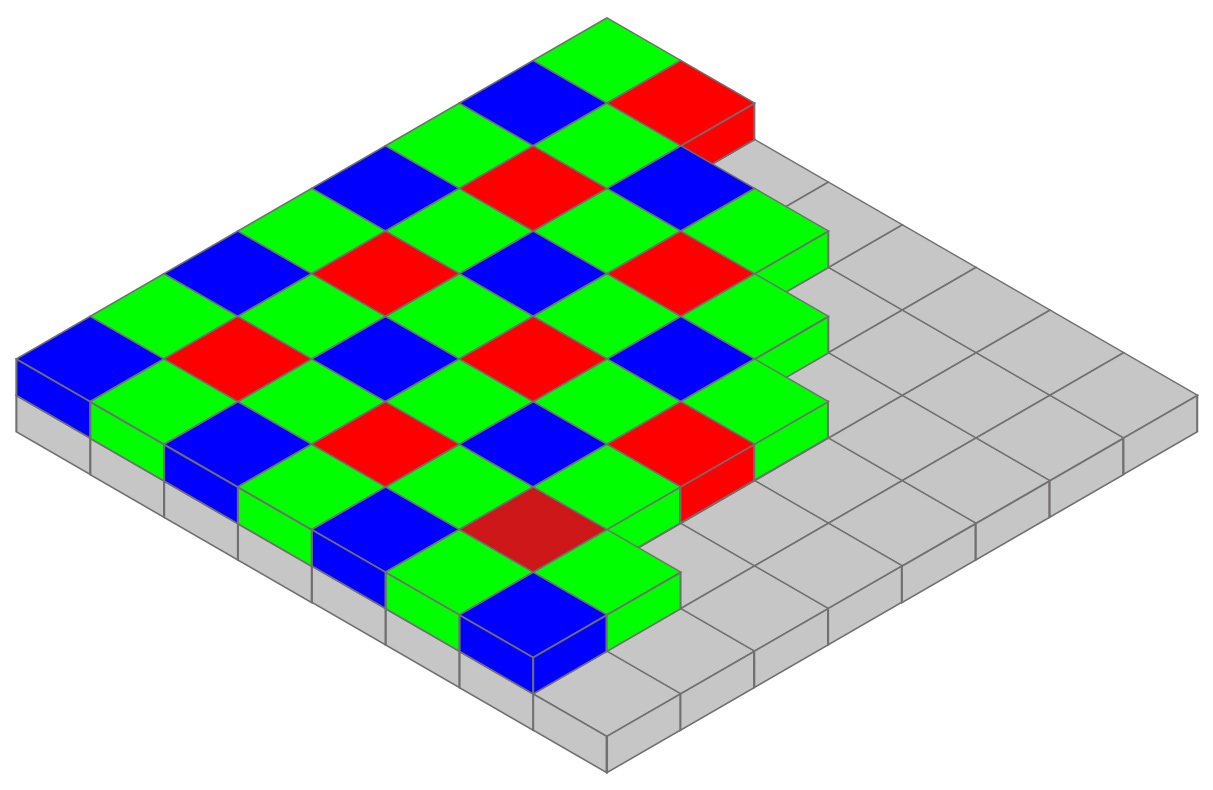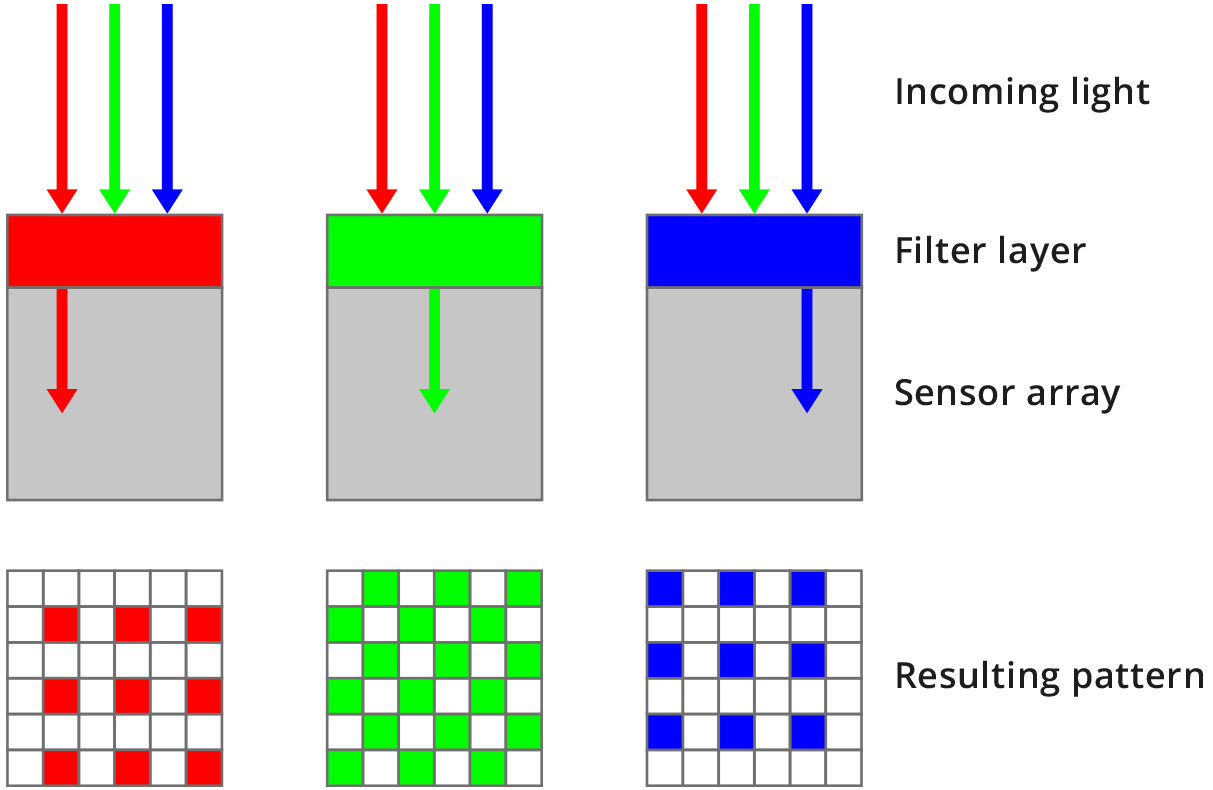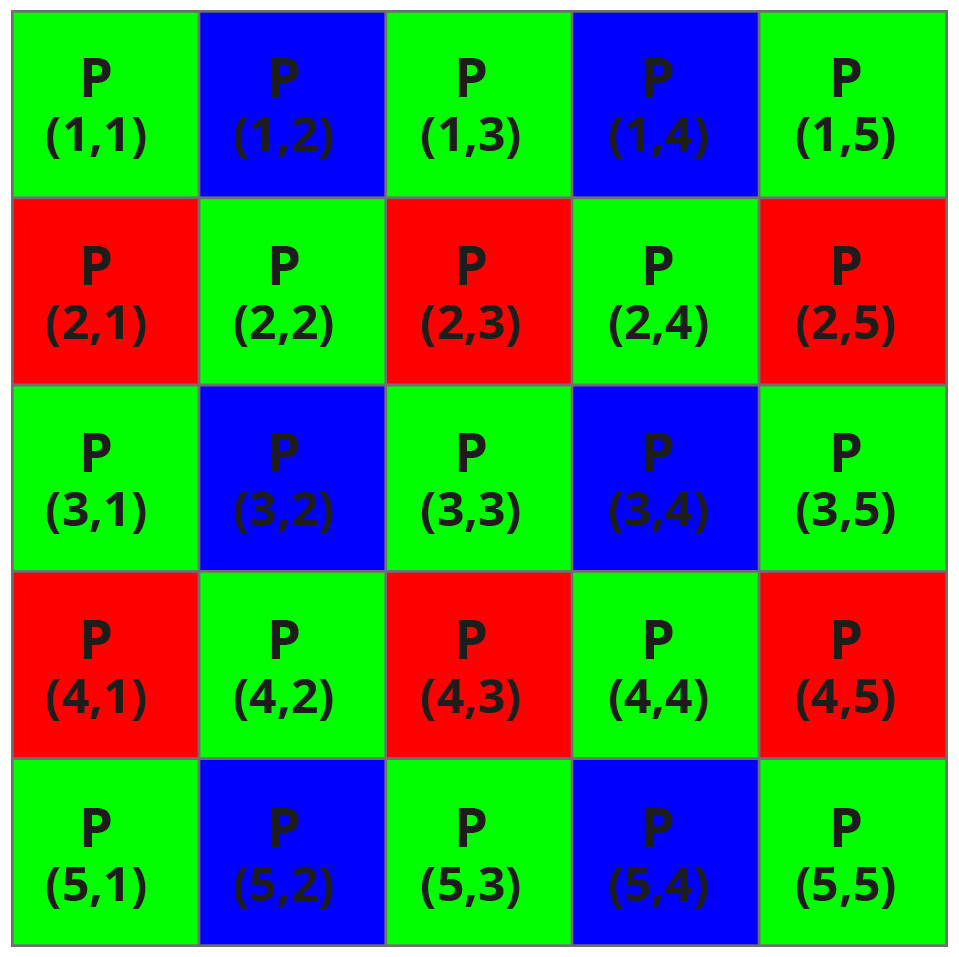## Monochrome VS color sensors

After the acquisition process, every pixel of the image provides a grey level information: typically the quantization is based on 256 grey levels (if the resolution is 8 bit), 1024 levels (10 bit) or 4096 (12 bit).

Thus, the image obtained is monochrome (black and white).

To display a color image, at least 3 coordinates are required: a red coordinate, a green coordinate and a blue coordinate. These 3 values can be expressed in the same way of a monochrome image, with a range of 256, 1024 or 4096 levels for all the 3 channels.

In the following example some colors are represented:

• White (R=255, G=255, B=255)
• Black (0,0,0)
• Green (0,255,0)
• Yellow (255,255,0)
• Purple (255,0,255)

In order to obtain the color information, two steps are necessary.The first step is to place above all the sensor array a color filter: the most common is the Bayer filter (see figure). Its typical pattern consists in a 50% of green pixel, 25% of red pixel and 25% of blue pixel, arranged as in the figure above.

For example in a green pixel only incoming rays whose wavelength is near 550 nm (i.e. green light) can pass through the filter layer and can be absorbed by the sensor (see the lower figure).

Rays with other wavelength are rejected.

Once the charge has been collected, the second step is to reconstruct the color information for all the pixel: this operation is called demosaicing (or debayering).

For example, pixel P(3,2) has only the blue information, since its color filter is blue. To obtain also the red and green coordinates one possible choice is to compute the two values by avaraging the nearest red and green pixel.

In this case the red value will be equal to:

Red_(3,2)=(Red_(2,1)+Red_(2,3)+Red_(4,1)+Red_(4,3))/4

And the green value will be equal to:

Green_(3,2)=(Green_(2,2)+Green_(3,1)+Green_(3,3)+Green_(4,2))/4

With a large mask used for averaging, a more accurate color can be obtained, but, on the other side, the algorithm will be heavier and, consequently, the processing time will be longer.To sum up, what parameters should be considered when choosing between mono and color sensor?

• Color sensor is necessary when dealing with application in which the color information is important.

Obviously, if the application needs a color image, the only choice is to use a color sensor (for example, inspection and quality control applications).

• Color sensor are intrinsically slower.

While for a monochrome sensor each pixel is usually described by 8 bits, for a color sensor each pixel has 8 bit for the red channel, 8 bit for the green channel and 8 bit for the blue channel, so three times the number of data to be processed, resulting in a higher processing time and, consequently, a slower frame rate.

• Monochrome sensor can achieve a higher resolution.

In a color camera, the presence of a Bayer filter can contribute to decrease the performance in terms of optical resolution of the system. Furthermore, also the demosaicing algorithm can introduced errors in color reconstruction.

Hence, if the color information is not necessary, a monochrome sensor should be preferred.

Next →